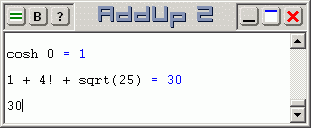# Scientific Calculations with AddUp 2

Scientific calculations with AddUp are performed by entering complex series of numbers and operators to form the expression to evaluate, as well as various math functions. The expression can be typed in and edited at will, then pressing the Enter key causes it to be evaluated. There is no need to use buttons with this calculator, everything that needs to be evaluated can be directly entered in the work area.Available mathematical operators are:

• Subtraction: -
• Multiplication: *
• Division: /
• Percent: % (for example, 5% = 0.05)
• Factorial: ! (for example, 5! = 120)
• Power (exponent): either ^ or ** (for example, 3^2 = 9)

Parentheses can also be used to nest sub-expressions. For example:
1 + (2 * 3) = 1 + 6 = 7
(1 + 2) * 3 = 3 * 3 = 9

Scientific functions are numerous. They include the mathematical functions that are normally found on a scientific calculator: square root, cubic root, power, exponent, logarithm (in various bases), factorial, etc.

Trigonometric functions are included: sine, cosine, tangent, cotangent, secant and cosecant. As well, inverse trigonometric functions are included: arc-sine, arc-cosine, arc-tangent, arc-cotangent, arc-secant and arc-cosecant.

Akin to trigonometric functions, hyperbolic functions are also provided: hyperbolic sine, hyperbolic cosine, hyperbolic tangent, hyperbolic cotangent, hyperbolic secant and hyperbolic cosecant. As well, inverse hyperbolic functions are included: hyperbolic arc-sine, hyperbolic arc-cosine, hyperbolic arc-tangent, hyperbolic arc-cotangent, hyperbolic arc-secant and hyperbolic arc-cosecant.

For more advanced math and engineering, complex numbers are natively supported. Complex number functions include: real and imaginary extractors, polar conversion, argument (or phase angle), complex conjugate, norm (or magnitude) and square of the norm. Complex numbers can be used directly in most operations where they would normally apply.

More basic arithmetic functions include: absolute value, inverse, modulo and remainder functions, percent and signum.